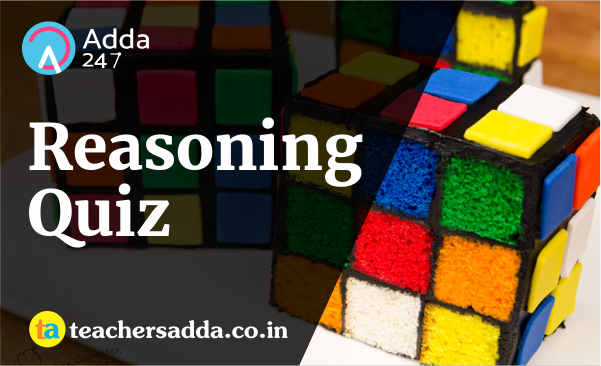The reasoning is the most scoring section in any competitive exam. It also develops or nourishes our critical thinking skills as compared to other subjects. In DSSSB,KVS Exam, As a student to score well in this part, you have to start your preparation now because there is negative marking as well. The more you have prepared, the better you score.

Q1. In the following question, select the number which can be placed at the sign of question mark (?) from the given alternatives.(a) 189
(b) 227
(c) 277
(d) 339

Q2. How many triangles are there in the given figure?(a) 8
(b) 10
(c) 12
(d) 14

Q3. In the following question below are given some statements followed by some conclusions. Taking the given statements to be true even if they seem to beat variance from commonly known facts, read all the conclusions and then decide which of the given conclusion logically follows the given statements.

Statements:
I. All rackets are bats.
II. All bats are wickets.
Conclusions:
I. Some wickets are rackets.
II. All wickets are rackets.

(a) Only conclusion (I) follows.
(b) Only conclusion (II) follows.
(c) Neither conclusion (I) nor conclusion (II) follows.
(d) Both conclusions follow.

Q4. From the given options, which figure can be formed by folding the figure given in the question?Q5. Identify the diagram that best represents the relationship among the given classes.

Staff, Manager, WorkerQ6. Which answer figure will complete the pattern in the question figure?Q7. From the given answer figures, select the one in which the question figure is hidden/embedded.Q8. A piece of paper is folded and punched as shown below in the question figures. From the given answer figures, indicate how it will appear when opened?Q9. If a mirror is placed on the line AB, then which of the answer figure is the right image of the given figure?Q10. A word is represented by only one set of numbers as given in any one of the alternatives. The sets of numbers given in the alternatives are represented by two classes of alphabets as shown in the given two matrices. The columns and row of Matrix-I are numbered form 0 to 4 and that of Matrix-II are numbered from 5 to 9. A letter from these matrices can be represented first by its row and next by its column, for example, 'X' can be represented by 21, 44, etc, and 'R' can be represented by 67, 98, etc. Similarly, you have to identify the set for the word "CREEP"(a) 10, 79, 23, 32, 42
(b) 24, 55, 14, 41, 12
(c) 33, 86, 32, 13, 43
(d) 42, 98, 41, 00, 34